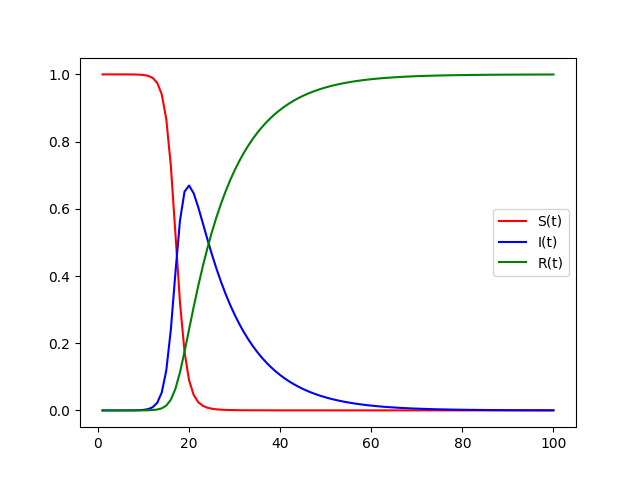# Graphing The SIR Model With PythonGraphing and solving simultaneous differential equations to model COVID-19 spread

If one good thing has come out of the COVID-19 pandemic, it’s the vast amount of data we have acquired. In light of technological advancements, we have access to more information and computing power, which we can use to predict and curb the spread of the virus. One of the simplest ways to do this is through the SIR model.

The SIR is a compartmental model that categorizes a _constant _population into three groups, namely the susceptible, infected, and recovered. These can all be expressed as functions that take time as an argument.

S(t) — The number of people who are susceptible to the disease

I(t) — The number of people infected with the disease

R(t) — Those incapable of infecting others; either recovered or diseased (hence a better name for this group may be “removed”)

Of note, S(t) + I(t) + R(t) = N at any time t, where N is the total population.

## Importing the needed Python libraries

``````from scipy.integrate import odeint

import numpy as np
import matplotlib.pyplot as plt``````

There are several libraries that we can use to smoothen out the calculations and graphing process. The SciPy library contains calculation methods that are “user-friendly and efficient.” We will be using their numerical integration function for this program. We will also be using Numpy for float step values and Matplotlib for graphing.

## Basic Data Types in Python | Python Web Development For Beginners

In the programming world, Data types play an important role. Each Variable is stored in different data types and responsible for various functions. Python had two different objects, and They are mutable and immutable objects.

## Data Visualization With Python | Data Visualization | Python For Data Science

🔥To access the slide deck used in this session for Free, click here: https://bit.ly/GetPDF_DataV_P 🔥 Great Learning brings you this live session on 'Data Vis...

## Data Visualization With Python: Matplotlib

Data visualization is the graphical representation of data in a graph, chart or other visual formats. It shows relationships of the data with images.

## Data Visualization in Data Science

How to use graphs effectively while working on Analytical problems. Data visualization is the process of creating interactive visuals to understand trends, variations, and derive meaningful insights from the data. Data visualization is used mainly for data checking and cleaning, exploration and discovery, and communicating results to business stakeholders.

## Step-by-step guide to Data Visualizations in Python

Create great-looking professional visualizations in Python using Matplotlib, Seaborn and much more packages. In this article, we are going to use some of Python’s well-known visualization packages, Matplotlib and Seaborn.